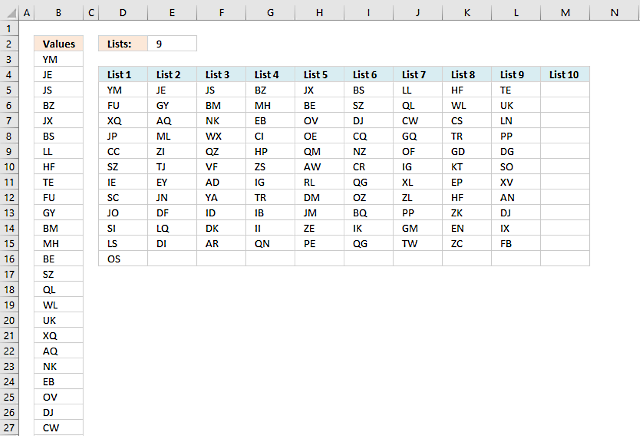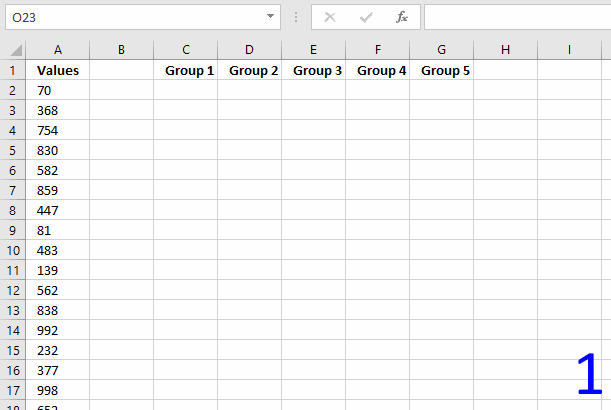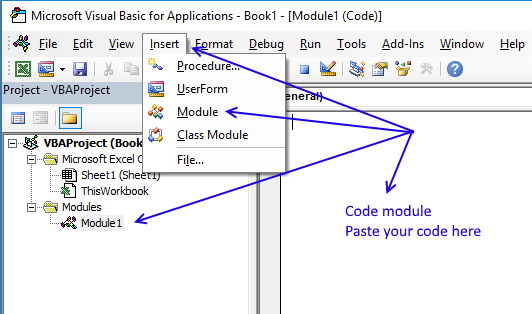Author: Oscar Cronquist Article last updated on January 31, 2019Question: How do I divide values equally into groups (3 lists or less)?

This post shows you two different approaches, an array formula, and a User Defined Function. You will find the UDF later in this post.

The above picture shows you values in column A and they are equally split across 9 columns, column D to M.

Formula in D5:

=IFERROR(IF(\$E\$2>=COLUMNS(\$A\$1:A1),INDEX(\$B\$3:\$B\$102,(ROWS(\$A\$1:A1))*\$E\$2-(\$E\$2-COLUMNS(\$A\$1:A1))),""),"")

Now copy cell D5 and paste to cells below and to the right.

### Explaining formula in cell D5

#### Step - 1 Keep track of lists

The COLUMNS function works just like the ROWS function except it counts columns instead of rows in a cell reference.

 Cell COLUMNS function Result D5 COLUMNS(\$A\$1:A1) 1 E5 COLUMNS(\$A\$1:A2) 2 F5 COLUMNS(\$A\$1:A3) 3

Cell E2 contains the number of lists to put the values into.

\$E\$2>=COLUMNS(\$A\$1:A1)

becomes

9>=1

and returns TRUE. This makes the formula return blank cells when the number of lists are greater than the value in cell E2.

#### Step 2 - Return blanks if all lists are populated

The IF function lets you specify a value if the logical expression returns TRUE (argument 2) and another value if FALSE (argument 3).

IF(\$E\$2>=COLUMNS(\$A\$1:A1),INDEX(\$B\$3:\$B\$102,(ROWS(\$A\$1:A1))*\$E\$2-(\$E\$2-COLUMNS(\$A\$1:A1))),"")

becomes

IF(TRUE,INDEX(\$B\$3:\$B\$102,(ROWS(\$A\$1:A1))*\$E\$2-(\$E\$2-COLUMNS(\$A\$1:A1))),"")

and returns

INDEX(\$B\$3:\$B\$102,(ROWS(\$A\$1:A1))*\$E\$2-(\$E\$2-COLUMNS(\$A\$1:A1)))

#### Step 3 - Return number based on an expanding cell reference

The ROWS function returns the number of rows in a cell reference, this cell ref is special. It expands as the formula is copied to cells below.

ROWS(\$A\$1:A1)

returns 1.

 Cell ROWS function Result B3 ROWS(\$A\$1:A1) 1 B4 ROWS(\$A\$1:A2) 2 B5 ROWS(\$A\$1:A3) 3

#### Step 4 - Multiply with cell E2

ROWS(\$A\$1:A1)*\$E\$2

becomes

1*9 equals 9.

#### Step 5 - Subtract \$E\$2 with COLUMNS

\$E\$2-COLUMNS(\$A\$1:A1))

becomes

9-1 equals 8

#### Step 6 - Calculate row number

ROWS(\$A\$1:A1)*\$E\$2-(\$E\$2-COLUMNS(\$A\$1:A1))

becomes

9-8 equals 1.

#### Step 6 - Get value based on row number

The INDEX function returns a value in cell range based on a row and column number. This is a single column cell ref so the column number is not neccessary in this case.

INDEX(\$B\$3:\$B\$102,(ROWS(\$A\$1:A1))*\$E\$2-(\$E\$2-COLUMNS(\$A\$1:A1)))

becomes

INDEX(\$B\$3:\$B\$102,1)

and returns "YM" in cell D5.

### Get the Excel filesplit-values-into-groups-using-excel-formulav3.xlsx

### User defined functionYou decide how many groups you want by selecting a cell range with as many columns as you want groups and then enter the UDF. It is designed to group values depending on how many columns you have selected before entering it.

The animated picture above shows you a cell range with 5 columns.

This post shows you how to categorize values into worksheets:

Recommended articles

Rearrange values based on category [VBA]
In this post I am going to rearrange values from a list into unique columns. Before: After: The code Get […]

and this post shows you how to categorize values to columns based on a condition:

Recommended articles

How to enter an array formula

1. Select cell range C2:G11
2. Paste above array formula to your formula bar3. Press and hold CTRL and SHIFT keys simultaneously
4. Press Enter once

The formula in the formula bar now looks like this: {=GroupValues(A2:A24)}
Don't enter these curly parentheses yourself, they appear automatically if you did the above steps correctly.

Recommended articles

A beginners guide to Excel array formulas
Array formulas allows you to do advanced calculations not possible with regular formulas.

VBA code

```Function GroupValues(rng As Range)
Dim result As Variant
c = Application.Caller.Columns.Count
r = Application.Caller.Rows.Count
ReDim result(1 To r, 1 To c)
i = 1
For ro = 1 To r
For co = 1 To c
If rng.Cells(i) <> "" Then
result(ro, co) = rng.Cells(i)
Else
result(ro, co) = ""
End If
i = i + 1
Next co
Next ro
GroupValues = result
End Function
```

How do I copy the code to my workbook?

1. Open VB editor (Alt+F11)
2. Insert a module to your workbook
3. Paste code to code module4. Go back to Excel
Note, make sure you save your workbook as a *.xlsm file or your workbook will lose all your vba code next time you close it.

### Get the Excel fileGroup-values.xlsm